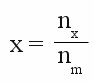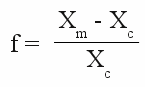# Observational error

## Measuring

A unit of measurement is a definite magnitude of a physical quantity, hence a a physical property of a body or substance. The value of a physical quantity is expressed as the product of a numerical value and the unit of measurement. Metering means building the ratio between the physical quantity and the unit of measurement:

[8.1]Where is:
x - result of measurement (numerical value), nx - value of a physical quantity, nm - unit of measurement

If a rod is 2 meter long, it is twice as long as the unit of measurement called "meter", the ratio is 2:1. I am 1.91m tall (long), hence the International Prototype Meter must be multiplied with the numerical value 1.91, to get the same length.
Like explained at the chapter measurement, different units are used for different physical properties. Many units can be transfered into each other, for example the unit "centimeter" is a hundredth of a meter and speed is the quotient of length unit to time unit, but a length unit can't be transferred directly into a time unit.

## Comparing

Figure 1:
To measure means comparing a physical quantity with the unit of measurement. Let's have a closer look at the procedure of comparing the mass of different objects, which is shown at the drawing to the left:
The mass of a mammoth is determined with the help of stones. The used measuring instrument is a seesaw, which can be adjusted horizontally whenever the absolute value of the acting torque at the left side equals those of the right side and they are pointing into contrarious directions. Let's assume that the distance between the mammoth and the center of rotation equals those of the deposited stones and the big stone below the timber (which is the fulcrum). One basic requirement is that the mass of all deposited stones is as close as possible to those of the unit "1 Stone" which is specified as the unit of measurement. Until these days, the international prototype kilogram defines the base unit of mass and is used to determine the weight or mass of other objects.

## Uncertainty

Let's have a look at possible sources of errors of the weighing machine above:
1.) The mass of each deposited stone has to be adjusted to those of the unit "1 Stone". Those adjustment is called calibration.
2.) The distance between the mammoth's center of mass and the fulcrum must equal those between the fulcrum and the center of mass of all deposited stones which is hard to put into practice especially while determining the mass of a living mammoth. If one half of the timber is noticeable longer than the other one, a systematic error will be the consequence. Such an error leads to a variation of the resulting values in such a way that it can be compensated by a correction factor. For example the determined value of the mammoth's mass will be lower than the real value if the animal is closer to the fulcrum than the deposited stones.
3.) The timber has to be adjusted horizontally to be able to determine the balanced condition. Reading errors are inevitable, because the arrangement will always move slightly.
4.) By using stones, the mass of the mammoth can be determined as an integer multiple of the unit " 1 Stone". It will be hard to find a mammoth whose mass will meet this condition exactly. The arrangement above makes it possible to determine that the mass of the mammoth is between 5 and 6 stones. By using half stones, the value can be determined more precisely.
5.) If we would use a longer timber, the torque of a single stone increases because of the longer lever acting on the left side. The mammoth's lever, hence the acting torque on the right side is increasing, too. The same number of stones has to be deposited at the seesaw to balance the arrangement, but there is a difference: If an additional stone is put on the left side, the timber starts moving faster and we can use quarter or eighth stones to determine the mammoth's mass. Those precision of a measuring arrangement is called sensitivity.

## Deviations

The value detected by a measurement arrangement with no observational error is called correct value (Xc. Practical experience shows that there are errors at any measuring device and you alway get a value Xm which differs from Xc (except fom a fluke). It is important to know the maximum difference between Xm and Xc. Those error is given as absolute error or absolute deviation:

[8.2]     F = Xm - Xc

Where is:
F - absolute error, Xm - detected value, Xr - correct value

or as relative error respectively relative deviation:

[8.3]f - relative deviation, Xm - detected value, Xr - correct value

The maximum deviation between a detected value and the true value is called error limit. The definite magnitude of the unit "kilogram" and so it's true value is the "international prototype kilogram". If the absolute error limit of a weighing scale is 0.1kg, the true value Xt is somewhere between 5.1kg and 5.3kg if the detected value is 5.2kg and the value is noted as: 5,2kg * (1 ± 0,1). It doesn't make sense to equip those weighing instrument with a scale displaying two decimal places or to estimate however those second decimal.

## 1 is never exactly 1

At the following chapters we will learn that the complexity of a measurement increases, the lower the required error limit should become. You can always get closer to the true value, but it is impossible to reduce the observational error to zero. If we observe the "international prototype kilogram", those platinum-iridium cylinder has to be cleaned from visible defilements if the absolute error limit is set to 0.1kg. While reducing those value to 0.001kg, we have to avoid the condensation of humidity at the surface of the cylinder. Below that limit, chemical reactions between the prototype and the ambient atmosphere have to be inhibited, the temperature and the barometric pressure has to be considered and so on...